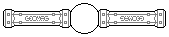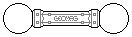Web textodigital.com
Around the rhombicosidodecahedron, 2
If we add twelve regular icosahedra to the rhombicosidodecahedron, as we did in Around the rhombicosidodecahedron, 1, but we build then twelve regular dodecahedra on top, twenty intersecting icosidodecahedra fit exactly among them. The finished object would have this look:Twelve icosahedra, twelve dodecahedra, and twenty icosidodecahedra around a rhombicosidodecahedron 2174 pieces: 542 balls, 1356 rods, 276 pentagons (11.86 kg)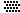If, instead of building the whole object, we do without an icosahedron, a dodecahedron, and five icosidodecahedra, we can place a pentagonal foot: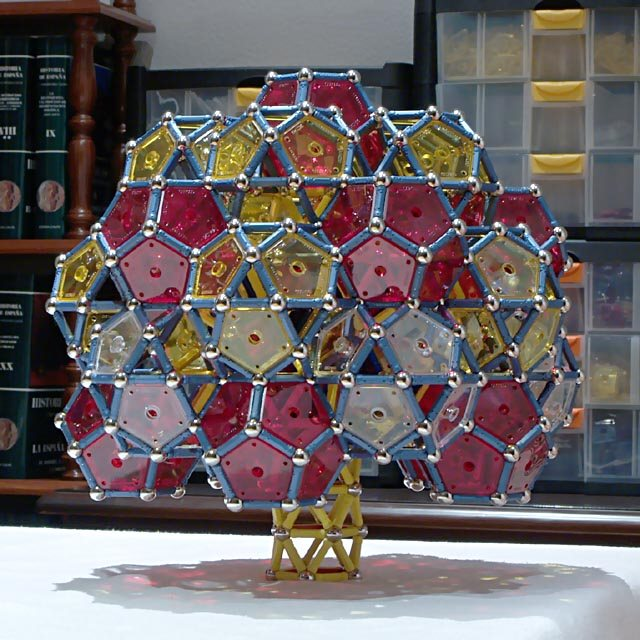Eleven icosahedra, eleven dodecahedra, and fifteen icosidodecahedra around a rhombicosidodecahedron 1945 pieces: 486 balls, 1216 rods, 243 pentagons (10.62 kg)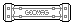In the next construction, we don't add objects trying to fill the space. We seek, instead, a big surface around the rhombicosidodecahedron, in a similar way as when building the rhombitruncated icosidodecahedron. As this surface will not be able to support the weight of the object, we begin by building a strong foot for supporting the core rhombicosidodecahedron. Then, we build the protruding structures which will hold the surface, about the same as the branches of a tree hold its crown: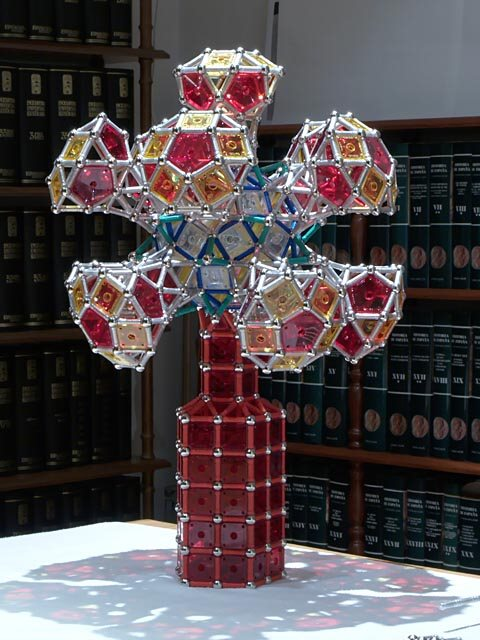«Tree» around a rhombicosidodecahedron, without «leaves»
Around each «branch» we place ten pentagons, of which five share a vertex with one of each of the surrounding branches. In the following pictures, these sharing pentagons are red, the intermediate ones being yellow: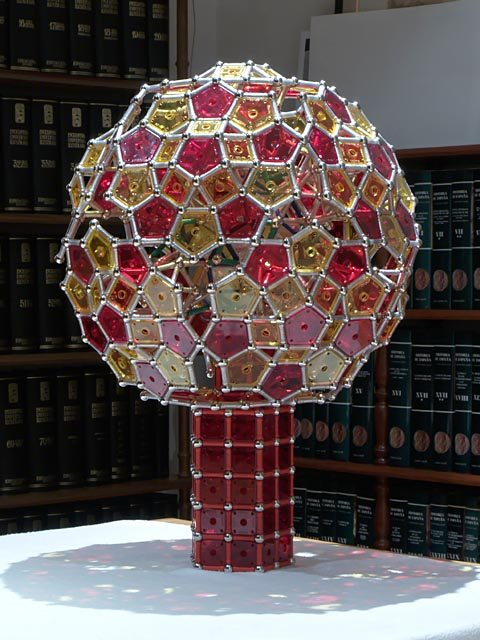«Tree» around a rhombicosidodecahedron, side view 2994 pieces: 739 balls, 1858 rods, 221 pentagons, 176 squares (16.07 kg)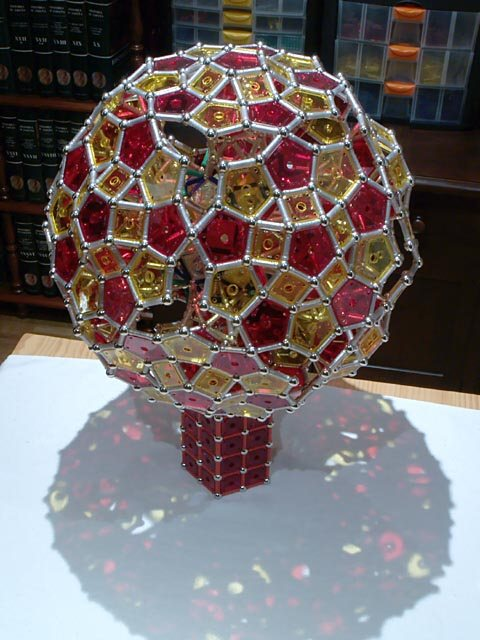«Tree» around a rhombicosidodecahedron, oblique top view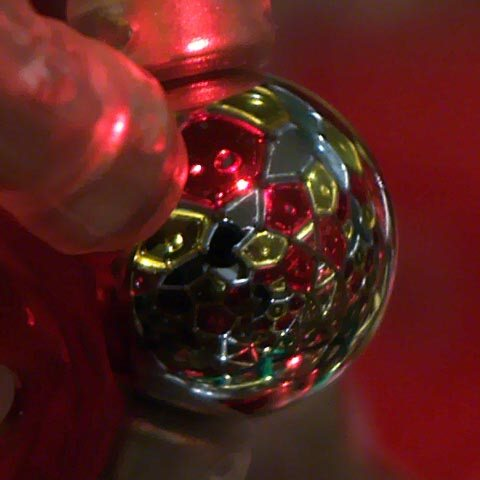«Tree» around a rhombicosidodecahedron, detail